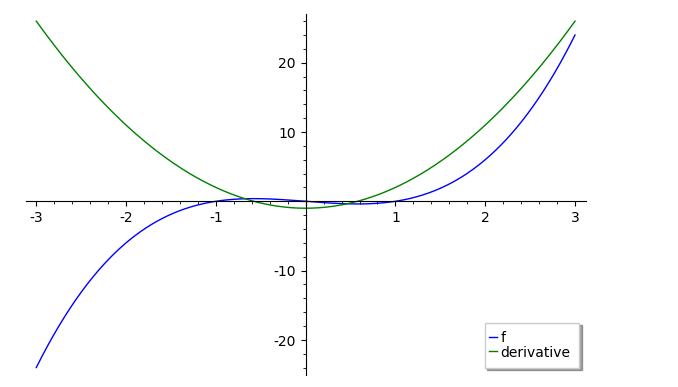# plotted real intersection but solve only shows imaginary

I plotted x^3 - x and its derivative 3*x^2 - 1 to get two intersections in the real plane.

plot([x^3-x,3*x^2 - 1],-3,3,color=['blue','green'],legend_label=["f","derivative"])However, when I solved the two with solve(x^3-x == 3*x^2 - 1, x), all I get are imaginary values.

solve(x^3-x == 3x^2 - 1,x)

[x == -1/2*(1/9*I*sqrt(37)*sqrt(3) + 1)^(1/3)*(I*sqrt(3) + 1) - 2/3*(-I*sqrt(3) + 1)/(1/9*I*sqrt(37)*sqrt(3) + 1)^(1/3) + 1, x == -1/2*(1/9*I*sqrt(37)*sqrt(3) + 1)^(1/3)*(-I*sqrt(3) + 1) - 2/3*(I*sqrt(3) + 1)/(1/9*I*sqrt(37)*sqrt(3) + 1)^(1/3) + 1, x == (1/9*I*sqrt(37)*sqrt(3) + 1)^(1/3) + 4/3/(1/9*I*sqrt(37)*sqrt(3) + 1)^(1/3) + 1]


Shouldn't solve show me the two intersections or am I disastrously confused?

edit retag close merge delete

I see the graph didn't show as an attachment. What am I doing wrong there?

Congratulations on formatting the code block!!

You can also format the inline portions of code with backticks.

This way, the * will show as * instead of being interpreted as begin-italics and end-italics.

I see the graph didn't show as an attachment. What am I doing wrong there?

How did you try to attach the graph? Ok, it seems repaired now.

Sort by » oldest newest most voted

When solving the roots of a 3rd degree polynomial, sometimes the real solutions are expressed in terms of the imaginary unit for instance when using The Cubic Formula : "Cardan's formula has the drawback that it may bring such square roots into play in intermediate steps of computation, even when those numbers do not appear in the problem or its answer. For instance, consider the cubic equation x^3-15x-4=0. (This example was mentioned by Bombelli in his book in 1572.) That problem has real coefficients, and it has three real roots for its answers. But if we apply Cardano's formula to this example, we use a=1, b=0, c=-15, d=-4, and we find that we need to take the square root of -109 in the resulting computation. Ultimately, the square roots of negative numbers would cancel out later in the computation".

If you provide the ComplexDoubleField to compute an approximation of the roots, here is what you get:

sage: p = (x^3-x) - (3*x^2 - 1)
sage: CDF
Complex Double Field
sage: p.roots(ring=CDF)
[(-0.6751308705666456, 1), (0.4608111271891108, 1), (3.2143197433775406, 1)]
sage: p.roots(ring=CDF, multiplicities=False)
[-0.6751308705666456, 0.4608111271891108, 3.2143197433775406]

more

The output of solve is a list of solutions in the form of equations x == ...:

sage: sol = solve(x^3 - x == 3*x^2 - 1, x)


Get the value of each solution as the right-hand side ("rhs"):

sage: a, b, c = [s.rhs() for s in sol]


Or use solution_dict=True to get solutions in dictionary form:

sage: sol = solve(x^3 - x == 3*x^2 - 1, x, solution_dict=True)


Then each solution s is a dictionary of the form {x: ...} and one can get its value as s[x]:

sage: a, b, c = [s[x] for s in sol]


In each case, we get:

sage: a
-1/2*(1/9*I*sqrt(37)*sqrt(3) + 1)^(1/3)*(I*sqrt(3) + 1) - 2/3*(-I*sqrt(3) + 1)/(1/9*I*sqrt(37)*sqrt(3) + 1)^(1/3) + 1
sage: b
-1/2*(1/9*I*sqrt(37)*sqrt(3) + 1)^(1/3)*(-I*sqrt(3) + 1) - 2/3*(I*sqrt(3) + 1)/(1/9*I*sqrt(37)*sqrt(3) + 1)^(1/3) + 1
sage: c
(1/9*I*sqrt(37)*sqrt(3) + 1)^(1/3) + 4/3/(1/9*I*sqrt(37)*sqrt(3) + 1)^(1/3) + 1


so it looks as though the solutions are non-real, since they involve I.

This is because there are well-known formulas to express solutions of cubic equations using radicals, sometimes involving square roots of negative numbers even when the final result is real.

Indeed, the results are real here, as we can check with the numerical_approx method (n for short):

sage: a.n()
0.460811127189111
sage: b.n()
-0.675130870566646 - 1.11022302462516e-16*I
sage: c.n()
3.21431974337754 - 5.55111512312578e-17*I


The numerical approximation is imperfect, and leaves a tiny imaginary component (e-16 means *10^-16) which however is only there due to rounding errors.

To get the results directly as exact algebraic numbers, and see directly whether or not they are real, and what their decimal expansion looks like, work with polynomials and their roots method rather than with the symbolic ring and the solve function.

sage: x = polygen(ZZ)
sage: p = x^3 - x
sage: q = p - p.derivative()
sage: q
x^3 - 3*x^2 - x + 1


The method roots will show (root, multiplicity) pairs:

sage: q.roots(AA)
[(-0.6751308705666461?, 1), (0.4608111271891109?, 1), (3.214319743377535?, 1)]


unless one specifies multiplicities=False:

sage: q.roots(AA, multiplicities=False)
[-0.6751308705666461?, 0.4608111271891109?, 3.214319743377535?]
`
more

Understood. .n().real() seems the easiest route. Msoft math just gives the real roots. At times, using Sagemath is using a Ferrari when a bicycle would do. But at least you learn more ;)